Open in App
Not now

# Frequency Distribution in R ggplot2

• Last Updated : 11 Oct, 2022

The ggplot2 package is a powerful and widely used package for graphic visualization. It can be used to provide a lot of aesthetic mappings to the plotted graphs. This package is widely available in R. The package can be downloaded and installed into the working space using the following command :

`install.packages("ggplot2")`

The ggplot method can be used to create a ggplot object. The graphical object is used to create plots by providing the data and its respective points. The data can be plotted using both points as well as lines.

Syntax: ggplot(data, aes = )

Arguments :

• data – The data to be plotted
• aes – The aesthetic mappings

The frequency table in R indicates the number of observations against each observed value either in discrete form or the total number of observations that lie within the specified interval ranges.

The geom_freqpoly() method can be used to add the frequency counts of the computed values. It can be added as a component in the geom object. It constructs a frequency polygon of the vector or data frame column that is specified. The geom_freqpolu() method has the following syntax :

Syntax: geom_freqpoly(mapping = NULL, data = NULL, bins = )

Arguments :

mapping – Aesthetic mappings

data – Data frame

bins – Number of bins to be plotted

## R

 `library``(ggplot2)`   `# creating a data frame ` `data_frame <- ``data.frame``(col1 = ``c``(1,3,5,6,23,6,2,5,7,16,8,9,36,7,` `                                  ``12,1,6,4,14,23,19,18,14,2,20,30))` `print``(``"Original Data"``)` `print``(data_frame)` ` `  `# creating frequency distribution with binwidth=10 ` `ggplot``(data_frame, ``aes``(x = col1)) +` `          ``geom_freqpoly``(bins=10)`

Output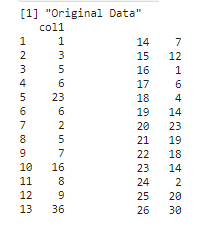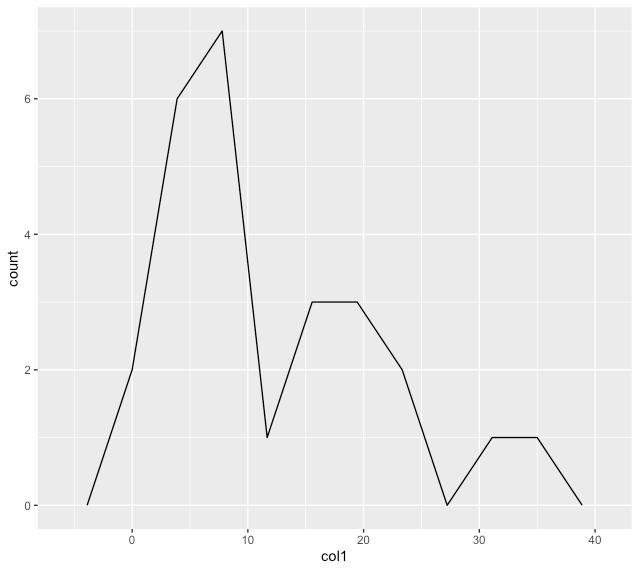Multiple columns of a data frame can also be used to construct the frequency distribution plots in R. The ggplot() method allows the user to customise the graphs by specifying the aesthetic mappings depending on the groups to which the values belong to using the colour and fill parameters respectively.

`ggplot (data , aes(x , colour = , fill)`

The following code snippet illustrates the usage of different coloured lines for values belonging to different groups. Since, there are three different grps “a”, “b” and “c”, three lines are computed on the graph. The frequencies are plotted for a unique set of values created in both col1 and grp columns respectively.

## R

 `library``(ggplot2)` `# creating a data frame `   `data_frame <- ``data.frame``(col1 = ``c``(1,3,5,6,23,6,2,5,7,16,8,9,36,7,12,` `                                  ``1,6,4,14,23,19,18,14,2,20,30,32,11,18,6),` `                         ``grp = ``c``(``rep``(``c``(``"a"``,``"b"``,``"c"``),10)))` `print``(``"Original Data"``)` `print``(data_frame)`     `# creating frequency distribution with ` `# different labeling for different grp values` `ggplot``(data_frame, ``aes``(x = col1, colour = grp, fill = grp)) +` `          ``geom_freqpoly``(bins=10)`

Output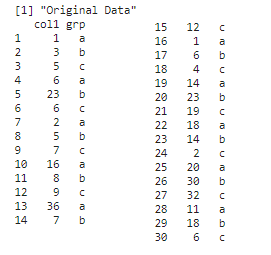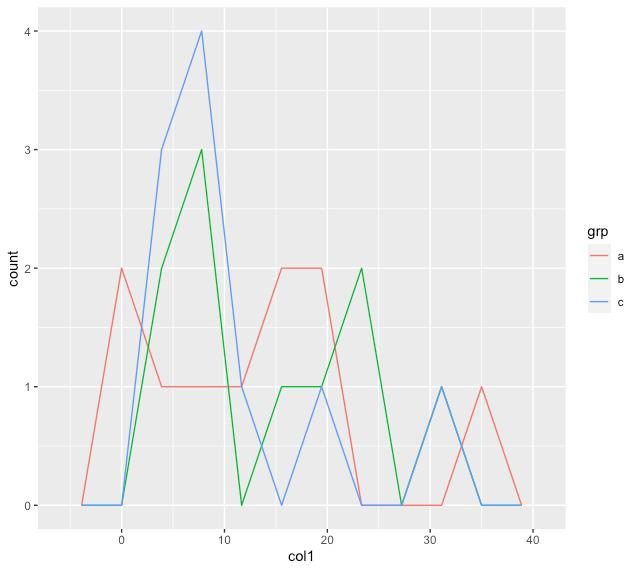The values belonging to different groups can be assigned to different facets in R. Facets are created to enhance the understandability of the program output. The number of facets are equivalent to the number of different groups in the data frame column. The facet_grid() can be added as a component to the ggplot object. It takes the respective column of the data frame to use as an argument of the method.

`facet_grid(df-col)`

The following code snippet creates three different facets for three grps, “a” , “b” and “c” and their frequency counts are plotted in their respective facets.

## R

 `library``(ggplot2)` `# creating a data frame `   `data_frame <- ``data.frame``(col1 = ``c``(1,3,5,6,23,6,2,5,7,16,8,9,36,7,12,` `                                  ``1,6,4,14,23,19,18,14,2,20,30,32,11,18,6),` `                         ``grp = ``c``(``rep``(``c``(``"a"``,``"b"``,``"c"``),10)))` `print``(``"Original Data"``)` `print``(data_frame)`     `# creating frequency distribution with different` `# labeling for different grp values` `# creating different facets for different groups` `ggplot``(data_frame, ``aes``(x = col1, colour = grp, fill = grp)) +` `  ``geom_freqpoly``(bins=10)+` `  ``facet_grid``(~grp)`

Output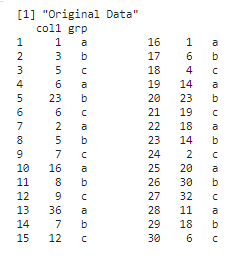My Personal Notes arrow_drop_up
Related Articles